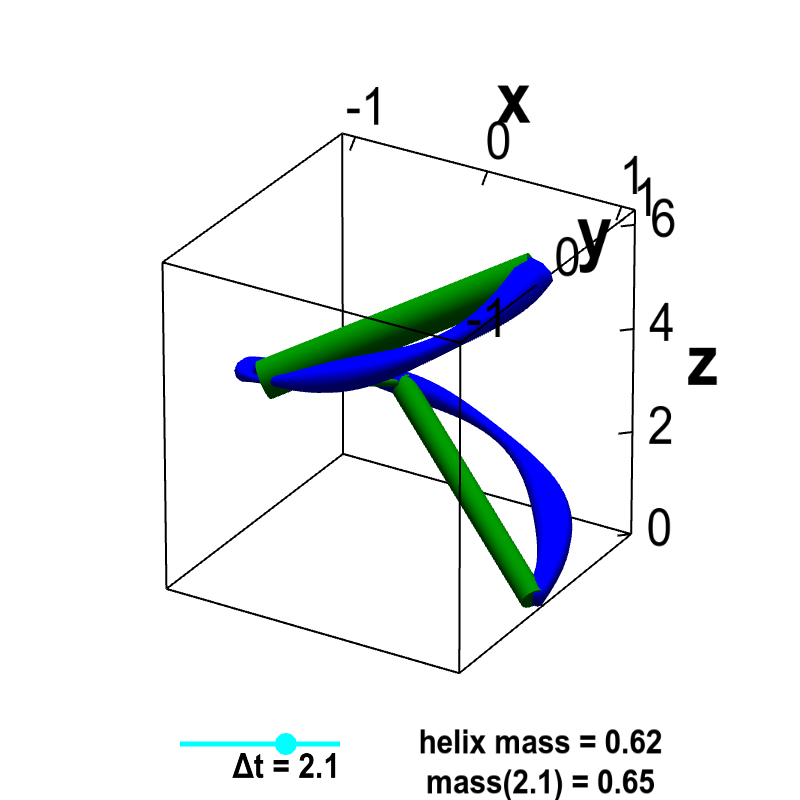# Math Insight

### Applet: Line integral of helix mass from densityThe function $\dllp(t) = (\cos t, \sin t, t)$, for $0 \le t \le 2\pi$ parametrizes a helix. The blue curve representing the helix is drawn with a variable width, representing the varying density $\dlsi(x,y,z)$ of the helix at each point $(x,y,z)=\dllp(t)$ along its length. The green line segments of constant width represent segments of constant density that approximate the helix. The discretization size of the line segments $\Delta t$ can be changed by moving the cyan point on the slider. As $\Delta t \to 0$, the mass of the line segment approximation, labeled mass($\Delta t$), approaches the actual mass of the helix.